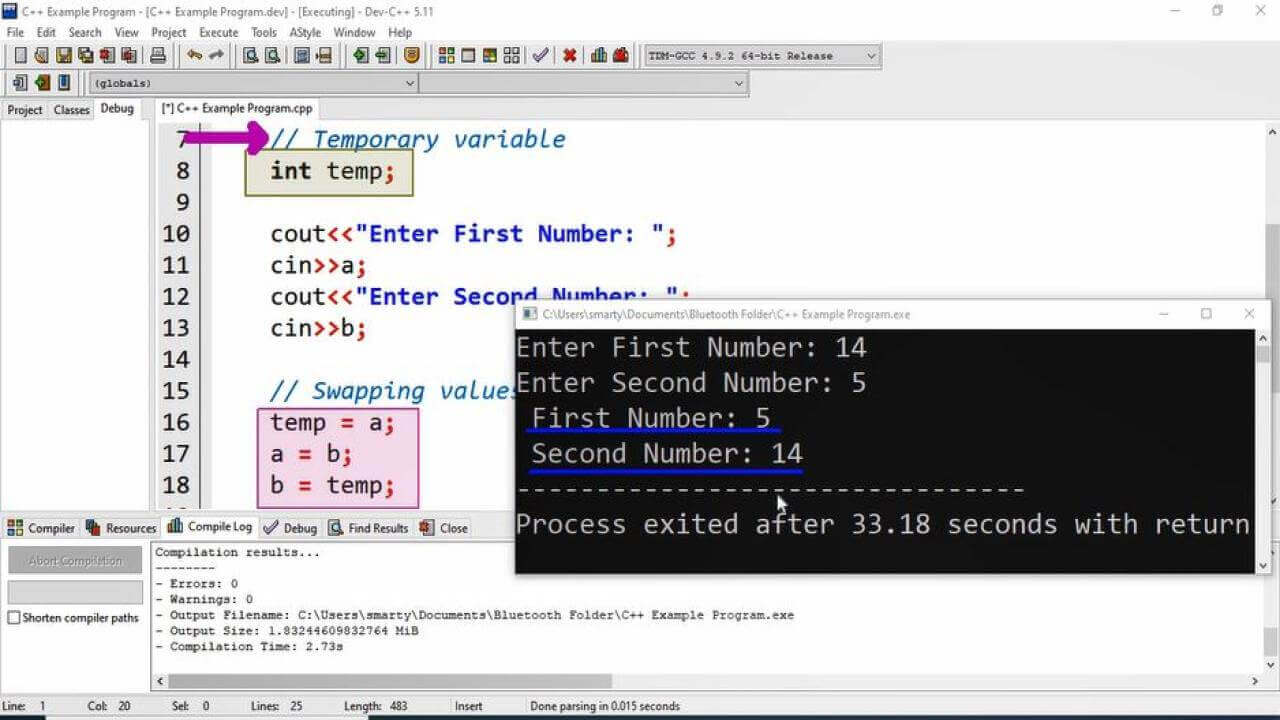# Input Two Numbers And Swap Their Values in C++

## Source Code

#include <iostream>

using namespace std;

int main(int argc, char** argv) {

// Variable Declaration for input
int a,b;
// Temporary variable
int temp;

cout<<"Enter First Number: ";
cin>>a;
cout<<"Enter Second Number: ";
cin>>b;

// Swapping values
temp = a;
a = b;
b = temp;

// Values after swapping
cout<<" First Number: "<<a;
cout<<"\n Second Number: "<<b;

return 0;
}

##Working

In this program first we declare three variables of type int. Then take input in variables a and b. We will not take input in temp variable. temp variable will used to temporarily store values of  variable a for interchange. After getting input we follow the following procedure.

• Assign value of variable a to temp variable
• Assign value of variable b to variable a (At this step variable a will contain value of variable b)
• Assign value of temp to b (At this step value of a will assign to variable b, because temp containing the value of  variable a)

After this swapping process program will print value of variable a and variable b.